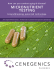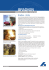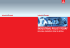Effect of solidification processing parameters on the cellular

Transcription

Effect of solidification processing parameters on the cellular
Journal of Alloys and Compounds 439 (2007) 114–127
Effect of solidification processing parameters on the cellular spacings
in the Al–0.1 wt% Ti and Al–0.5 wt% Ti alloys
M. Gündüz a,∗ , H. Kaya b , E. Çadırlı b , N. Maraşlı a ,
K. Keşlioğlu a , B. Saatçi a
a
Erciyes University, Faculty of Arts and Sciences, Department of Physics, Kayseri, Turkey
b Niğde University, Faculty of Arts and Sciences, Department of Physics, Niğde, Turkey
Received 3 July 2006; received in revised form 24 August 2006; accepted 26 August 2006
Available online 26 September 2006
Abstract
The aim of this work is to investigate the relationship between solidification processing parameters (temperature gradient, G, growth rate, V, and
cooling rate,Ṫ ) and cellular spacing, λ, in the Al–0.1 wt% Ti and Al–0.5 wt% Ti alloys grown at high growth rates. In order to achieve this aim, the
specimens were solidified under argon atmosphere and steady-state conditions upward with different temperature gradients, G at a constant growth
rate, V and with different V at a constant G for the Al–Ti alloys in the Bridgman type directional solidification apparatus. The cellular spacing,
λ was measured and expressed as functions of solidification processing parameters, G, V and Ṫ by using a linear regression analysis. The results
were compared with similar previous experimental work and current theoretical models suggested for cellular growth.
Keywords: Al–Ti alloys; Microstructure; Directional solidification; Cellular spacing; Crystal growth
1. Introduction
1.1. Solidiﬁcation of single phase and peritectic alloys
Solidification of single phase alloys and peritectic growth
have been studied [1–5] for about 50 years. Recently peritectic
solidification has attracted more attention in experimental and
theoretical studies [6–36]. Since many technologically important materials are peritectics, studies on unidirectional solidification of peritectic alloys have been carried out on such
as Zn–Ag [24,25], Sn–Cd [26–28], Pb–Bi [29–33], Zn–Cu
[11,34,35], Sn–Sb , high temperature superalloys Al–Ti
[16,19], Ni–Ti  and Ni–Al , superconducting materials
YBCO [14,15], magnetic materials Fe–Nd–B , and structural materials Fe–Ni [17,18].
Peritectic microstructures are characterized by the growth
competition between α primary and a peritectic β phase, which
can both coexist with the liquid at the peritectic temperature Tp
(Fig. 1). In case of single growth of the primary phase (Co1 ) inter-
∗
Corresponding author. Tel.: +90 352 4374937x33126; fax: +90 352 4374933.
E-mail address: [email protected] (M. Gündüz).
doi:10.1016/j.jallcom.2006.08.246
face temperature of the primary phase decreases towards TLα in
order to achieve the steady-state growth with planar interface.
On the other hand when peritectic alloy directionally solidified
under planar growth conditions, the rejection of solute in the liquid leads to the formation of a solute boundary layer . The
composition in the liquid at the interface increases as the singlephase solidification and the interface temperature decreases with
time until a steady-state growth condition is achieved at the
solidus temperature of the alloy. The three phase peritectic reaction (L + β → α) cannot occur at Tp but must occur below Tp .
However, below Tp α can also form directly by crystallization
from liquid and unless the temperature is maintained constant
all the α can be produced this way . Once α forms, a three
phase interface is produced where the liquid is CLα and hence the
β is unstable and may dissolve to bring the liquid composition
back to CLP (Fig. 1). As soon as this happens the liquid becomes
undercooled with respect to α and α will grow consuming the
excess B atoms. When the temperature fallen from TL to TP ,
liquid remote from the β crystals is richer in component B than
CLP , because compositions gradients must be established while
B atom are withdrawn from the melt to feed the growing β crystals . Although the peritectic reaction is normally isothermal,
the initial reaction L + β → α is stilled by forming of an envelope
M. Gündüz et al. / Journal of Alloys and Compounds 439 (2007) 114–127
Fig. 1. Hypothetical peritectic phase diagram, showing symbols used for phases,
compositions and temperatures (after ).
115
of solid α around each β particle. Further transformation then
occurs by diffusion through the peritectic envelope while temperature falls below TP . At any given temperature TR (Fig. 1) the
α envelope will separate β phase of composition Cβ from liquid
composition CL but through the thickness x, of the composition will vary between Cα1 and Cα2 (Fig. 1). Thus, dissolution of
the β phase by diffusion of the β component through the envelope is assisted by a concentration gradient (Cα1 − Cα2 )/x, the
magnitude of which is depend on the relative slopes of the Cα1
and Cα2 lines .
In peritectic systems two-phase morphologies have been
observed under the condition close to the limit of constitutional
undercooling, such as peritectic banding [26,34,41–43] oscillation [33,42,43] and peritectic coupled growth [44–47]. Simultaneous (coupled) growth of two phases with planar solid/liquid
interface has been suggested to occur in peritectic alloys close to
the peritectic temperature, similar to eutectic alloys [1,26,48,49].
Coupled growth characterized as a co-operative growth mechanism so that simultaneous growth of two phases from the melt
occurs. Coupled growth is characterized by a macroscopically
that composite front in which the two phases grow together
parallel to the growth direction with either lamellar or rod mor-
Fig. 2. Partial Al–Ti phase diagram (after ) and microstructures observed for Al–0.1 wt% Ti and Al–0.5 wt% Ti alloys.
116
M. Gündüz et al. / Journal of Alloys and Compounds 439 (2007) 114–127
phology. Cellular peritectic coupled growth is only possible
when both phases are morphologically stable and the liquid
between the cells and dendrites becomes richer in B atoms .
criterion. Trivedi model gives
λ = 2.83[LΓm(ko − 1)D]0.25 Co0.25 G−0.5 V −0.25
(Trivedi; cellular/dendritic)
1.2. Directional solidiﬁcations, solidiﬁcation processing
parameters and cellular spacings
In directional solidification experiments, solidification processing parameters, temperature gradient G and growth rate V
can be independently controlled so that one may study the dependence of microstructural parameter (λ) on either G at constant V
or V at constant G for the constant initial solute composition Co .
Most experimental studies have shown that the microstructural
parameters decrease as solidification processing parameters (G,
V and Ṫ ) increase, for the constant Co . The above observations
have led, over the past decades, to be the establishment of relationships between the solidification processing parameters and
microstructural parameters. A literature survey shows several
theoretical models [51–53] and numerical models [54–57] used
to examine the influence of solidification processing parameters (G, V, Co ) on the microstructural parameter, although the
agreement between theory and experiment was still in complete
.
Hunt , Kurz and Fisher  and Trivedi  have been
proposed detailed theoretical models to characterize cells and
primary dendrite spacings during steady-state growth conditions, as a function of G, V and Co . Hunt attempted to allow
for the interaction of the diffusion fields between neighboring
cells using an approach suggested by Bower et al. . The relationship between solidification processing parameters (G, V, Co )
and λ for a cellular and dendritic front with the growth condition is determined by the minimum undercooling. The Hunt 
model gives,
(3)
where L is a constant that depends on the harmonic perturbations.
These theoretical models are very similar at high growth rate for
λ and the difference among them is a constant only. Warren and
Langer [60,61] proposed a solution of this problem that rather
than a unique value of λ there may be an allowable range of
primary spacings for any given set of solidification conditions.
However, The Warren and Langer model is unable to account for
the ability of a dendritic array to decrease its primary spacings
by tip splitting or higher-order side branching in response to a
change in solidification conditions. Hunt and Lu  and Kurz
et al. [56,57] have proposed numerical models to characterize
cells and primary dendrite spacings during steady-state growth
conditions, as a function of V and Co .
Hunt and Lu  have proposed an analytical expression for
corresponding cellular spacing from their numerical predictions
of the lower limit of stable cellular/dendritic spacing and is represented by the following equations,
0.41
Γko
−0.745
λ = 4.09ko
D0.59 V −0.59
mCo (ko − 1)
(Hunt and Lu; cellular)
(4)
λ = 2.83[Γm(ko − 1)D]0.25 Co0.25 G−0.5 V −0.25
(Hunt; cellular/dendritic)
(1)
where m is liquidus slope, k is partition coefficient, D is diffusion
coefficient in liquid and Γ is Gibbs–Thomson coefficient.
Kurz and Fisher  assumed that the shape of the cell
or dendrite fully developed, including side branches can be
approximated as an ellipsoidal revolution and using the marginal
stability criterion for an isolated dendrite or cell and they simplified their results for the low velocity (V < Vcs /k) and for the high
velocity (V > Vcs /k) regimes (where Vcs is the critical velocity at
which the planar interface becomes unstable). For V > Vcs /k they
obtained as follows.
0.25
Γm(ko − 1)D
λ = 4.3
Co0.25 G−0.5 V −0.25
2
kO
(Kurz and Fisher; cellular/dendritic)
(2)
The other theoretical model to characterize λ as function of G,
V, Co was developed by Trivedi . Trivedi model is a result of
the modification of the Hunt model, and used marginal stability
Fig. 3. The schematic illustration of the cellular spacings measurements transverse and longitudinal sections (a) transverse section; (b) longitudinal section;
(c) schematic illustration of the microstructure; (d) triangle method for cellular
spacing; (e) area counting method for cellular spacing.
M. Gündüz et al. / Journal of Alloys and Compounds 439 (2007) 114–127
117
Table 1
The experimental results and relationships between the cellular spacings and solidification processing parameters for Al–0.1 wt% Ti alloy
Al–0.1 wt% Ti alloy system
Solidification parameters
Cellular spacings
G (K/mm)
V × 10−3
3.8
5.1
6.2
7.3
9.1
9.1
9.1
9.1
9.1
8.2
8.3
8.3
8.4
8.3
16.6
42.8
81.0
166.0
(mm/s)
.
(␮m)
±
±
±
±
±
±
±
±
±
98.9
84.4
74.4
68.0
62.6
53.9
40.6
34.4
27.4
T (K/s)
0.034
0.045
0.052
0.061
0.070
0.133
0.327
0.745
1.300
95.2
83.0
77.4
66.8
61.1
51.0
38.3
32.2
27.2
2.9
2.4
2.1
1.9
1.7
2.0
2.9
1.2
1.8
Constant (k)
(␮m0.49
The relationships
λ∗∗
tr
λ*
λ∗∗
ar
(␮m)
±
±
±
±
±
±
±
±
±
8.2
11.3
9.2
7.5
4.5
5.8
5.0
4.3
2.7
(␮m)
107.1
90.5
80.2
71.3
65.8
56.7
43.5
36.6
29.1
±
±
±
±
±
±
±
±
±
8.7
13.2
11.1
6.6
6.2
6.1
5.1
4.1
2.2
Correlation coefficients (r)
λ* = k1 G−0.51 ,
λ* = k2 V−0.27 ,
λ∗ =
−0.32
k3 (Ṫ )
,
=
λ∗∗
a
k4 G−0.54 ,
λ∗∗
a =
k5 V −0.27 ,
λ∗∗
a =
−0.32
k6 (Ṫ )
r1 = −0.989
r2 = −0.994
r3 = −0.984
r4 = −0.994
r5 = −0.994
r6 = −0.984
K0.51 )
k1 = 5.62
k2 = 107.2 (␮m1.27 s−0.27 )
k3 = 27.5 (␮m K0.32 s−0.32 )
k4 = 4.83 (␮m0.46 K0.54 )
k5 = 114.8 (␮m1.27 s−0.27 )
k6 = 28.8 (␮m K0.32 s−0.32 )
λ* : The values of the cellular spacing measured from the longitudinal section of the samples; λ∗∗
tr : the values of the cellular spacing measured from the transverse
section of the samples using by the triangle method; λ∗∗
ar : the values of the cellular spacing measured from the transverse section of the samples using by the area
counting method; and λ∗∗
a : average values of cellular spacing measured from the transverse section of the samples. Bold values are used to emphasize the increase
of G at a constant V, and also, increase of Vat a constant G.
A good approximation for λ at high growth velocity is given by
Kurz et al. [56,57] as,
ΓDko
λ = 4π
mCo (ko − 1)
0.5
V −0.5
(Kurz et al.; cellular)
(V > Vcs ) and the experimental results were compared with
the results obtained by the theoretical models. Also several
experimental studies in the literature [62–70] have compared
with these theoretical predictions under steady-state growth
conditions, for alloys of different systems: Pb–Sb [62,63],
succinonitrile–acetone [64,65], Al–Cu [66,67], Al–Zn [68,69],
Sn–Pb , and a agreement between theory and experiment is
generally reported.
(5)
The theoretical models for high growth rate were chosen
because the experiments were carried out for high growth rates
Table 2
The experimental results and relationships between the cellular spacings and solidification processing parameters for Al–0.5 wt% Ti alloy
Al–0.5 wt% Ti peritectic alloy system
Solidification parameters
G (K/mm)
V × 10−3
5.1
6.3
7.6
8.9
10.3
10.3
10.3
10.3
10.3
8.4
8.3
8.2
8.4
8.3
16.6
43.0
83.0
165.0
Constant (k)
(␮m0.47
Cellular spacings
(mm/s)
.
The relationships
(␮m)
λ∗∗
tr
±
±
±
±
±
±
±
±
±
96.1
82.6
75.9
70.4
64.7
56.2
44.8
36.7
30.5
T (K/s)
λ*
0.039
0.048
0.058
0.066
0.079
0.165
0.407
0.790
1.45
94.5
80.1
75.4
69.0
64.0
53.3
43.9
35.1
26.8
2.6
3.0
2.7
2.9
2.1
2.2
2.1
1.8
1.9
(␮m)
±
±
±
±
±
±
±
±
±
4.8
5.0
5.3
4.5
4.3
4.0
2.7
2.2
2.1
Correlation coefficients (r)
K0.53 )
k7 = 5.62
k8 = 117.5 (␮m1.28 s−0.28 )
k9 = 30.9 (␮m K0.31 s−0.31 )
k10 = 5.50 (␮m0.46 K0.54 )
k11 = 112.2 (␮m1.25 s−0.25 )
k12 = 33.9 (␮m K0.29 s−0.29 )
r7 = −0.989
r8 = −0.989
r9 = −0.989
r10 = −0.994
r11 = −0.994
r12 = −0.989
Bold values are used to emphasize the increase of G at a constant V, and also, increase of Vat a constant G.
λ∗∗
ar
(␮m)
103.5
97.5
89.8
76.2
67.4
57.9
45.8
39.4
34.3
±
±
±
±
±
±
±
±
±
6.1
5.2
4.5
4.6
4.7
4.4
3.0
2.9
2.0
λ* = k7 G−0.53 ,
λ* = k8 V−0.28 ,
λ∗ =
−0.31
,
k9 (Ṫ )
∗∗
λa =
k10 G−0.54 ,
λ∗∗
a =
k11 V −0.25 ,
λ∗∗
a =
−0.29
k12 (Ṫ )
118
M. Gündüz et al. / Journal of Alloys and Compounds 439 (2007) 114–127
Fig. 4. Variation of cellular spacings of the directionally solidified Al–0.1 wt% Ti alloy, (a1 ) longitudinal section, (a2 ) transverse section (G = 3.8 K/mm, V = 8.2 ␮m/s);
(b1 ) longitudinal section, (b2 ) transverse section (G = 6.2 K/mm, V = 8.3 ␮m/); (c1 ) longitudinal section, (c2 ) transverse section (G = 9.1 K/mm, V = 8.3 ␮m/s); (d1 )
longitudinal section, (d2 ) transverse section (G = 9.1 K/mm, V = 42.8 ␮m/s); (e1 ) longitudinal section, (e2 ) transverse section (G = 9.1 K/mm, V = 166 ␮m/s).
The aim of this work is to obtain more insight into the
microstructure and phase selection of the single phase and the
peritectic growth and to investigate the relationship between
solidification processing parameters (G, V, Ṫ ) and cellular spacing, in the Al–0.1 wt% Ti and Al–0.5 wt% Ti alloys grown at
high growth rates. In the Al rich Al–Ti alloys, solute pile up
at growing interface does not cause convection because density
of Ti is larger than that of Al. Therefore the Al–Ti system was
selected as a model system to investigate the cellular structure
in peritectic alloys. Al rich Al–Ti alloy is excellent alloy system
to study coupled growth for three main reasons: first, in contrast to many other peritectic systems, the mushy zone range is
M. Gündüz et al. / Journal of Alloys and Compounds 439 (2007) 114–127
119
Fig. 5. Variation of cellular spacings of the directionally solidified Al–0.5 wt% Ti alloy, (a1 ) longitudinal section, (a2 ) transverse section (G = 5.1 K/mm, V = 8.4 ␮m/s);
(b1 ) longitudinal section, (b2 ) transverse section (G = 7.6 K/mm, V = 8.2 ␮m/); (c1 ) longitudinal section, (c2 ) transverse section (G = 10.3 K/mm, V = 8.3 ␮m/s); (d1 )
longitudinal section, (d2 ) transverse section (G = 10.3 K/mm, V = 43 ␮m/s); (e1 ) longitudinal section, (e2 ) transverse section (G = 10.3 K/mm, V = 165 ␮m/s).
small  (∼5 K) which values it convenient to carry out experiments (Fig. 2), secondly, the small density difference between
the solute and the solvent limits the amount of convection present
in the melt as compared to other alloy systems such as Cu–Zn,
Pb–Bi, Sn–Cd, thirdly, all necessary physical parameters related
to Al–Ti system are well known.
2. Experimental procedure
Al–Ti alloys containing 0.1 and 0.5 wt% Ti were prepared from high-purity
Al (99.99%) and Ti (99.99%). Bulk alloy of each composition was melted by
vacuum melting furnace. After allowing time for melt homogenization, molten
alloy was poured into the prepared 13 graphite crucibles (250 mm in length 4 mm
i.d. and 6.35 mm o.d.) in a hot filling furnace. Then each specimen was positioned
120
M. Gündüz et al. / Journal of Alloys and Compounds 439 (2007) 114–127
Fig. 6. Scanning electron micrographs of the directionally solidified Al–0.1 wt% Ti alloy (a1 ) longitudinal section, (a2 , a3 ) higher magnification views from the same
longitudinal section area; (b1 ) transverse section, (b2 ) higher magnification views from the same transverse section area (G = 9.1 K/mm, V = 8.3 ␮m/s).
in a graphite cylinder (300 mm in length 10 mm i.d. and 40 mm o.d.) in a Bridgman type furnace. After stabilizing the thermal conditions in the furnace under
an argon atmosphere, the specimen was grown by pulling it downwards at various constant rates by means of different speed synchronous motors. Specimens
were solidified under steady-state conditions with different G (3.8–9.1 K/mm)
at constant V (8.3 ␮m/s) and with different V (8.3–166 ␮m/s) at a constant G
(9.1 K/mm) for Al–0.1 wt% Ti alloy and with different G (5.1–10.3 K/mm)
at a constant V (8.3 ␮m/s) and with different V (8.3–165 ␮m/s) at a constant
G (10.3 K/mm) for Al–0.5 wt% Ti alloy (Tables 1 and 2). After 100–120 mm
steady-state growth of the samples, the samples were quenched by pulling them
rapidly into the water reservoir.
2.1. Measurement of temperature gradients, G growth rates, V
and cooling rates, Ṫ
The temperature of the Bridgman type furnace was controlled by a Pt/Pt-13%
Rh thermocouple placed between the heating element and the alumina tube. The
temperature could be controlled to about ±0.1 K during the run. The thermocouples were placed capillary alumina tubes (0.8 mm i.d., 1.2 mm o.d.) which were
positioned approximately 10 mm apart and parallel to the heat flow direction
inside the crucible. Throughout the experiment, temperature distribution was
obtained by measuring the temperature in the sample by three chromel/alumel
thermocouples (type-K). All the thermocouple leads were taken to an ice/water
cold junction, then to a WPA analog potentiometer and to a Kipp–Zonen chart
recorder capable of recording to 1 ␮V. (The experimental details are given in
.)
The thermocouples were, recorded simultaneously for measurement of the
temperature gradients on the solid/liquid interface in the liquid. When the
second thermocouple was at the solid–liquid interface and then third thermocouple in the liquid their temperatures were used to obtain the temperature gradient, G. The positions of the thermocouples were measured after
the quench. The cooling water level was always kept at the same position in
order to check the furnace temperature. Thus, the sample temperature was
constant. The temperature gradient can be altered by changing the temperature of the sample and/or the distance between the cooling water level and
the hot stage. G can be kept constant during the run by keeping the temperature of the cooler and the hotter part of the furnace and the distance between
them.
M. Gündüz et al. / Journal of Alloys and Compounds 439 (2007) 114–127
121
Fig. 7. Scanning electron micrographs of the directionally solidified Al–0.5 wt% Ti alloy (a1 ) longitudinal section, (a2 , a3 ) higher magnification views from the same
longitudinal section area; (b1 ) transverse section, (b2 ) higher magnification views from the same transverse section area (G = 10.3 K/mm, V = 8.3 ␮m/s).
The growth rate was calculated with two different methods. In the first
method, the values for the growth rate were calculated from the measurements
of the time taken for the solid/liquid interface to pass the thermocouples separated by a known distance. In the second method, the total solidification time
and solidification distance (on the longitudinal section of the polished sample)
were measured. The ratio of the distances to the times was measured to obtain
the growth rates and these were similar for both methods.
The cooling rate Ṫ was determined from the cooling curves obtained by
the Kipp–Zonen recorder during the directional solidification process of each
thermocouple. The values are given in Tables 1 and 2.
2.2. Metallographic analysis
The unidirectionally grown quenched specimens were removed from the
graphite crucible, then ground to observe the solid–liquid interface, and longitudinal (parallel to the growth direction) section, which included the quenched
interface, was separated from the specimen. Then the samples included quench
interface was cold-mounted with epoxy-resin. Thereafter, longitudinal and transverse sections of the samples were observed with standard metallographic
techniques. An etchant made up of 5-ml hydrofluoric acid, 5-ml nitric acid
and 95-ml water was used to provide a suitable contrast for Al–Ti alloys. The
microstructure of the specimen was investigated by the light microscope (Olympus, BH-2) and scanning electron microscopy (LEO-l).
2.3. Measurement of cellular spacings, λ
The λ values measured on the transverse sections gave more accurate results
than the λ values measured on the longitudinal section. Two different methods
were used for measurement of the cellular spacings on the transverse sections
(Fig. 3). The first method is the triangle method . The triangle occurred by
joining the three neighbor hexagonal centers and sides of the triangle corresponded to λ (Fig. 3d). In this method at least 200–300 cellular spacings λ∗∗
tr
122
M. Gündüz et al. / Journal of Alloys and Compounds 439 (2007) 114–127
values were measured for each specimen. The second method is the area counting method [73–76]. In this method, the average cellular spacing values, λ∗∗
ar
were calculated from,
λ∗∗
ar =
B
M
A 0.5
N
(6)
where B (1.075 for hexagonal structures) is correction factor, M the magnification
factor, A the total specimen cross-section area and N the number of hexagonal
on the cross-section. λ∗∗
ar values were measured on the cross-section, at least
on five different regions for each specimen in this method (Fig. 3e). λa values,
obtained from average values of the area counting method, λ∗∗
a and the triangle
method, λ∗∗
tr are given in Tables 1 and 2.
3. Result and discussion
A series of experiments were carried out at a constant G
(9.1 K/mm) with different V (8.3–166) ␮m/s and at a constant
V (8.3 ␮m/s) with different G (3.8–9.1 K/mm) for Al–0.1 wt%
Ti alloy and also at a constant G (10.3 K/mm) with different V
(8.3–165 ␮m/s) and at a constant V (8.3 ␮m/s) with different G
(5.1–10.3 K/mm) for Al–0.5 wt% Ti alloy. Figs. 3–7 show longitudinal and transverse views of the samples studied to examine
the effect of solidification processing parameters on the cellular
spacing. The measured cellular spacing with standard deviations
and relationships between cellular spacing and the solidification
processing parameters are given in Tables 1 and 2.
3.1. Effect of the temperature gradient on the cellular
spacing
The photographs of the unidirectionally solidified Al–Ti
alloys are shown in Figs. 4–7. In order to determine λ versus
G dependency, measurements of cellular spacings were made
over a range of temperature gradients at a constant velocity
(V = 8.3 ␮m/s) for Al–0.1 wt% Ti and Al–0.5 wt% Ti alloys.
The plots of the log λ versus log G data obtained at constant
Fig. 8. (a) Variation of cellular spacing λ, with temperature gradients G, at a constant growth rate (V = 8.3 ␮m/s); (b) variation of cellular spacing λ, with growth
rates V, at a constant temperature gradient (G = 9.1 K/mm); (c) variation of cellular spacing λ, with cooling rates Ṫ of the directionally solidified Al–0.1 wt% Ti alloy
system.
M. Gündüz et al. / Journal of Alloys and Compounds 439 (2007) 114–127
123
Fig. 9. (a) Variation of cellular spacing λ, with temperature gradients G, at a constant growth rate (V = 8.3 ␮m/s); (b) variation of cellular spacing λ, with growth
rates V, at a constant temperature gradient (G = 10.3 K/mm); (c) variation of cellular spacing λ, with cooling rates Ṫ of the directionally solidified Al–0.5 wt% Ti
alloy system.
velocity are shown in Figs. 8a and 9a for Al–0.1 wt% Ti and
Al–0.5 wt% Ti alloy systems, respectively. As can be seen from
Figs. 8a and 9a, a mathematical relationship can be described
between λ and G by linear regression analysis for each composition as
λ = k1 G−a
(7)
As can be seen from Figs. 8a and 9a, λ changed inversely
proportional to G. The exponent values (obtained from transverse section) of the temperature gradient equal to 0.56 and
0.57 for Al–0.1 wt% Ti and Al–0.5 wt% Ti alloys, respectively.
The exponent values of the temperature gradients for the longitudinal section equal to 0.52 and 0.50 for Al–0.1 wt% Ti and
Al–0.5 wt% Ti alloys, respectively. As can be seen from Table 3,
our exponent values (0.52–0.57) are in good agreement with
the values of 0.50, 0.50, 0.50, 0.50, 0.50 and (0.52 and 0.57)
obtained by Lapin et al.  for Ni–Al–Cr–Fr system, McCart-
ney and Hunt  for Al–0.63 at% Mg–1.39 at% Si system,
Eshalman et al.  for PWA1480 system, Young and Kirkwood  for Al–(2.4–10.1) wt% Cu systems, Taha  for
Al–(5.7–20) wt% Cu systems, Jin  for Fe–8 at% Ni system
and Tunca and Smith  for Zn–8 wt% Al system, respectively.
Furthermore, these experimental exponent values were found to
be very close to the value 0.50 predicted by Hunt  Kurz
and Fisher  and Trivedi  theoretical models for cellular
spacings.
3.2. Effect of the growth rate on the cellular spacing
Figs. 8b and 9b, Tables 1 and 2 show variation of λ with
V at the constant G (9.1 K/mm) for Al–0.1 wt% Ti alloy and a
constant G (10.3 K/mm) for Al–0.5 wt% Ti alloy. The variation
of λ on the log λ versus log V plot is essentially linear for the
growth rate ranges (8.3–165) ␮m/s. A linear regression analysis
124
M. Gündüz et al. / Journal of Alloys and Compounds 439 (2007) 114–127
Table 3
The experimental results for some metallic alloys in the literature
Composition
Range of G (K/mm)
Range of λ1 (␮m)
Range of V (␮m/s)
Relationship
–
–


Ref.
Al–(70–80 at% Ni) alloys
Zn–1.5–7.4Cu peritectic alloys
–
15
500–5000
20–4820
λ = 1200V−0.50
Zn–1.53–7.37Cu peritectic alloys
15
20–4820
λ1 = 310V−0.50
λ1 = 540V−0.59
3.5–103

500–4500
5–33
λ = 216V−0.50
–
2.8–8
10–120


8–490
λ1 = kG−0.30
λ1 = k2 V−0.23
120–750

λ1 = kV−0.38
λmin = 731.3V−0.375
λmax = 8674.1V−0.526
7.5–90

457–1141

λ1
(n = 0.32–0.71)
λ1 = kV−n (n = 0.29–0.40)
45.2–146.7

λ1 = kG−0.32
λ1 = kV−0.44
λ1 = 1.24 × 10−3 G−0.50 V−0.25
λ1 = 62G−0.50 V−0.25
λ1 = 102G−0.50 V−0.25
35.2–182

80–200
48–1270
167–195



132–630

160–500
77.5–850


–

4.3–16.3
144–859
–
80–200
–
–
120–750
66–118
80–225









Zn–3.37 wt% Cu peritectic
Fe–Ni peritectic alloys
–
9–10
Al–(3–24) wt% Cu
1.5–7.4
Al–4.5 wt% Cu
Al–0.53 wt% Zn
Al–0.26 wt% Mn
8.8
10–240
8.8
10–200
Pb–(5–95) wt% Sn
Pb–19 wt% Sn
Pb–8 wt% Au
Ni–Al–Cr–Fr
Al–0.63 at% Mg–1.39 at% Si
PWA1480
(Ni–12Ta–10Cr–5Co–5Al–4W–1.5Ti)
Al–2.4 wt% Cu
Al–4.4 wt% Cu
Al–10.1 wt% Cu
Al–(5.7–20) wt% Cu
Fe–8 at% Ni
Zn-6.5 wt% Al
Zn–8 wt% Al
Zn–11 wt% Al
Zn–8 wt% Al
Zn–(2.17) wt% Cu peritectic alloys
Fe–C–Mn–Si 0.09, 0.15C/1.4Mn/0.4Si
Al–40 wt% Cu
Pb–5.10 at% Sb
Al–11 wt% Mg
Al–(9.5–28.1) wt% Fe
Ni base superalloy (IN738LC)a
Pb–5.7 at% Sb
Fe–(3.5–4.5) at% Ni peritectic alloys
a
λ1 = (216–316)V−0.50
= kG−n
0.8–5.5
7–467
1.1–37
40
15
0.3–18.5
25.5
20
2–407
2.78–118
7.7–1000
100–233
5–13
2–9
11–42
7.5–10.5
7.5–12
50–500
7–430
4.1–102.8
10–1000
10–1000
λ1 = kG−0.50 V−0.50
λ1 = kG−0.50 V−0.36
λ1 = kG−0.50 V−0.43
λ1 = 55G−0.50 V−0.25
λ1 = 41G−0.50 V−0.25
λ1 = kV−m (m = 0.25–0.27)
λ1 = kV−m (m = 0.24–0.29)
7.5–12
10–1000
λ1 = kV−m (m = 0.25–0.30)
λ1 = kG−0.57
2.8–12
–
1.02–7.02
3
0.5–4.5
–
1–13
5–8.5
2–19.7
18
53–100
20–4800
33–340
4.2–42
50–120
–
10–6400
16–333
8–218
3–30
λ1 = kG−0.52
λ1 = 316V−0.50
λ1 = 53G−0.50 V−0.25
λ1 = kV−0.32
λ1 = 19G−0.50 V−0.25
λ1 = k(GV)−0.33
λ1 = kG−0.50 V−0.25
λ1 = 954(GV)−0.31
λ1 = 24G−0.50 V−0.25
–
Ni–(15.9Cr–8.1Co–3.3Ti–3.4Al–3W–1.9Mo–1.6Ta–0.8Nb–0.11C) wt%.
gives the proportionality equation as
λ = k2 V −b
(8)
Figs. 8b and 9b show clearly that an increase in V produce a
decrease in λ. The exponent value of the growth rate b is equal
to 0.26 and 0.24 for the Al–0.1 wt% Ti and Al–0.5 wt% Ti alloys,
respectively.
Decreasing of cellular spacings, λ with increasing growth
rates, V was determined by various workers for different alloy
systems [6,77,84,93–98]. The exponent values of the growth
rates, b values 0.40, 0.41 0.25, 0.50, 0.25, 0.23, 0.27, 0.54 and
0.26 obtained by Miyata et al.  for Al–Cu, Jesse and Giller
 for Cd–Zn, Sharp  for Al–Cu, Ma et al.  for Zn–Cu,
Yang et al.  for Cu–Mn, Lapin et al.  for Ni–Al–Cr–Fe,
Kloosterman and Hosson  for TiN, Feng et al.  for Al–Zn
and Pryds et al.  for Fe–Cr–C alloy, respectively. Our exponent values (0.26 and 0.24) is in good agreement with the values
0.25, 0.25, 0.23, 0.27, and 0.26 obtained by Sharp , Yang
et al. , Lapin et al. , Kloosterman and Hosson  and
Pryds et al. , respectively. These exponent values are in good
agreement with the value 0.25 predicted by Hunt , Kurz and
Fisher  and Trivedi  theoretical models. On the other
hand, these exponent values (0.26 and 0.24) are less than the
values of 0.40 and 0.41 obtained by Miyata et al.  and Jesse
and Giller  and also (0.59 and 0.50) predicted by Hunt and
Lu  and Kurz et al. [56,57] numerical models, respectively,
for cellular spacings. This might be due to the higher growth
rate exponent for the numerical models. On the other hand the
experimental λ values and the numerically obtained λ values
agree quite well for the growth rates between 15 and 150 ␮m/s.
M. Gündüz et al. / Journal of Alloys and Compounds 439 (2007) 114–127
125
Fig. 10. Comparison of cellular spacings, λ obtained with the experimental work and with the theoretical models [50,52,53] (a) for Al–0.1 wt% Ti alloy; (b) for
Al–0.5 wt% Ti alloy.
3.3. Effect of the cooling rate on the cellular spacing
Experimentally measured cooling rate (Ṫ ) values are given
in Tables 1 and 2 for Al–0.1 wt% Ti and Al–0.5 wt% Ti alloys,
respectively. As can be seen from Figs. 8c and 9c, the variations of λ versus Ṫ plot is essentially linear on the logarithmic
scale. Figs. 8c and 9c, Tables 1 and 2 show clearly that an
increase in cooling rate, Ṫ leads to a decrease in cellular spacings, λ. The linear regression analysis gives the proportionality
equation as,
λ = k3 Ṫ −c
(9)
The exponent value of the cooling rate c is equal to 0.33 and 0.32
for the Al–0.1 wt% Ti and Al–0.5 wt% Ti alloys, respectively.
Similar exponent values in the literature for different alloys 0.55,
0.25, 0.35, 0.36 were obtained by Rocha et al.  for Sn–Pb
alloys, David and Vitek , Gündüz and Çadırlı  for Al–Cu
alloys, by Çadırlı and Gündüz  for Pb–Sn alloys. These exponent values (0.33 and 0.32) are close to the values 0.25, 0.35 and
0.36 obtained by David and Vitek , Gündüz and Çadırlı 
and Çadırlı and Gündüz , respectively.
3.4. Effect of composition on the cellular spacing
As can be seen from Tables 1 and 2, the values of λ measured
from Al–0.5 wt% Ti alloy are higher than Al–0.1 wt% Ti alloy
for same G and V values (G = 5.1, 6.2, 7.3 K/mm; V = 8.3 ␮m/s).
This result is agreed with the theoretical models because accord-
Fig. 11. Comparison of cellular spacings, λ obtained with the experimental work and with the numerical models [57,59,60] (a) for Al–0.1 wt% Ti alloy; (b) for
Al–0.5 wt% Ti alloy.
126
M. Gündüz et al. / Journal of Alloys and Compounds 439 (2007) 114–127
ing to Eqs. (1)–(3) λ increase with the increasing composition,
Co . Furthermore, the exponent values of the growth rate (0.27)
and cooling rate (0.32) for Al–0.1 wt% Ti alloy are bigger than
the growth rate (0.25) and the cooling rate (0.29) for Al–0.5 wt%
Ti alloy.
3.5. The comparison of the experimental results with
theoretical models
The comparison of the experimentally obtained λ values with
the calculated λ values by the theoretical models (Hunt model
, the Kurz and Fisher model , the Trivedi model )
and numerical models (Hunt and Lu model  and Kurz et al.
model [56,57]) is given in Figs. 10 and 11. The thermophysical
parameters of Al–Ti alloys used in λ calculations for the models
are given in Appendix A. Fig. 10a and b shows the comparison of
the experimental cellular spacing, λ values with the theoretical
models [50,52,53] for Al–0.1 wt% Ti and Al–0.5 wt% Ti alloys,
respectively. As can be seen in Fig. 10a and b the calculated
line of λ with the Kurz–Fisher model and Trivedi model for
both compositions is fairly higher than the our experimental
values, but the calculated λ value with the Hunt model is in
good agreement with our experimental values. The numerical
model presented by Hunt and Lu  and Kurz et al. [56,57]
were also used to calculate values of λ as a function of V and
Co for constant temperature gradient. The comparison of our
experimental values of λ with calculated values of λ by Hunt
and Lu  and Kurz et al. [56,57] numerical models are given
in Fig. 11a and b. As can be seen in Fig. 11, our experimental
results are in quite good agreement with calculated values of
λ by Hunt–Lu model at high growth rates and by Kurz et al.
models at low growth rates for Al–0.1 wt% Ti and Al–0.5 wt%
Ti alloys.
Discrepancies are noticed between experimental and the calculated values for growth rates smaller than 15 ␮m/s and higher
than 150 ␮m/s.
4. Summary and conclusions
In the present study, Al–0.1 wt% Ti and Al–0.5 wt% Ti alloys
were solidified unidirectionally upwards under various solidification conditions. The formation of the cellular structure was
observed from the Al–0.1 wt% Ti and Al–0.5 wt% Ti alloys but
cell lengths relating to Al–0.5 wt% Ti composition are shorter
than Al–0.1 wt% Ti composition. The microstructural features
observed from the longitudinal and transverse views of the specimens studied for examining the influence of cellular spacing,
λ, depend on solidification processing parameters (G, V and Ṫ ).
The principal results can be summarized as follows.
1. Cellular spacings measured from the transverse sections,
λ∗∗
a are more reliable than the cellular spacings measured
from the longitudinal sections, λ∗a of the directionally solidified Al–0.1 wt% Ti and Al–0.1 wt% Ti alloys (Fig. 3 and
Tables 1 and 2).
2. It was seen that the values of λ decreases as the values of G, V
and Ṫ increase. The range of temperature gradients exponents
(a = 0.52–0.57) is good agreement with the previous experimental works [77–83]. The range of growth rate exponents
(b = 0.24–0.26) is very close to the previous experimental
works [6,77,95,96,98] and also the range of cooling rate
exponents (c = 0.32–0.33) are very close to previous experimental works [66,70,99].
3. As can be seen from Fig. 10a and b, the experimental λ values
are very close to results obtained by the Hunt model  but
the predicted λ values with the Kurz and Fisher  and the
Trivedi model  are fairly higher than our experimental λ
values.
4. The experimental λ values agree quite well with the values
obtained by Hunt and Lu  numerical model at high growth
rates and Kurz et al. [56,57] numerical models at low growth
rates.
5. As can be seen from Tables 1 and 2, λ values for the
Al–0.5 wt% Ti alloy are larger than the λ values for the
Al–0.1 wt% Ti alloy for same G and V values. This result
is also agreed with the theoretical models.
Acknowledgments
This research was financially supported by the The Scientific
And Technical Research Council of Turkey (TÜBİTAK Under
Contract No: MİSAG-125). Authors are grateful to TÜBİTAK
for their financial support.
Appendix A. Thermophysical parameters of Al rich
Al–Ti alloys
Property
Symbol
Unit
Value
Ref.
Melting point
Composition
Slope of liquid line
Gibbs–Thomson coefficient
Diffusion coefficient (liquid)
Distribution coefficient
The harmonic perturbations
Tm
Co
mL
Γ
DL
ko
L
K
wt%
K/wt%
␮m K
␮m2 /s
–
mJ/m2
938
0.1 and 0.5
46.62
0.131
2720
0.94
10





References
 M.C. Flemings, Solidification Processing, Mc Graw Hill, NewYork, 1974,
pp. 31–53.
 R. Elliott, Eutectic Solidification Processing—Cyrstalline and Glassy
Alloys, Butterworth, London, 1983, pp. 8–20.
 W. Kurz, D.J. Fisher, Fundamentals of Solidification, Trans Tech Publications, Aedermannsdorf, Switzerland, 1989, pp. 48–53.
 D.A. Porter, K.E. Easterling, Phase Transformation In Metals and Alloys,
Van Nostrand and Reinhold, New York, 1984, pp. 208–222.
 D.J. Verhoeven, Fundamentals of Physical Metallurgy, John Wiley &
sons, New York, 1975, pp. 245–264.
 A.B. Kloosterman, J.T.H.M. Hosson, J. Mater. Sci. 32 (1997) 6201–6205.
 H.W. Kerr, J. Cisse, G.F. Bolling, Acta Metall. 22 (1974) 677.
 K. Kaltenbach, S. Gama, D.G. Pinatti, K. Schulze, Z. Metallkunde 80
(1989) 511–514.
 W.J. Boettinger, S.R. Coriell, A.L. Greer, A. Karma, W. Kurz, M. Rappaz,
R. Trivedi, Acta Metall. 48 (2000) 43–70.
 D. Ma, Y. Li, S.C. Ng, H. Jones, Acta Metall. 48 (2000) 419–431.
 D. Ma, Y. Li, S.C. Ng, H. Jones, Acta Metall. 48 (2000) 1741–1751.
M. Gündüz et al. / Journal of Alloys and Compounds 439 (2007) 114–127
 H.P. Ha, J.D. Hunt, Metall. Trans. 31A (2000) 29.
 A. Das, I. Manna, S.K. Pabi, Acta Metall. 47 (1999) 1379–1388.
 C.J. Kim, Y.A. Jee, S.C. Kwon, T.H. Sung, G.W. Hong, Physica C 315
(1999) 263.
 B.A. Tent, D. Qu, D.L. Shi, W.J. Bresser, P. Boolchand, Z.X. Cai, Phys.
Rev. 58B (1998) 11761.
 R. Hermann, W. Loser, J. Appl. Phys. 83 (1998) 6399.
 O. Hunziker, M. Vandyoussefi, W. Kurz, Acta Metall. 46 (1998) 6325.
 A. Karma, W.J. Rappel, B.C. Fuh, Metall. Trans. 29A (1998) 1457.
 F. Meissen, P. Busse, in: J. Beech, H. Jones (Eds.), Solidification Processing 1997, University of Sheffield, UK, 1997, p. 460.
 H.W. Kerr, W. Kurz, Int. Mater. Rev. 41 (1996) 129.
 T. Umeda, T. Okane, W. Kurz, Acta Metall. 44 (1996) 4209.
 J.H. Lee, J.D. Verhoeven, J. Cryst. Growth 144 (1994) 353.
 O. Hunziker, W. Kurz, Acta Metall. 45 (1997) 4981.
 D.R. Uhlmann, G.A. Chadwick, Acta Metall. 9 (1961) 835.
 A. Ostowski, E.W. Langer, Proceedings of International Conference On
Solidification and Casting, Institute of Metals, London, 1977, p. 139.
 W.J. Boettinger, Metall. Trans. 5 (1974) 2023.
 H.D. Brody, S.A. David, Solidification and Casting of Metals, The Metals
Society, London, 1976, pp. 144–151.
 H.P. Ha, D.J. Hunt, Proceedings of International Conference On Solidification and Casting, Institute of Metals, London, 1977, p. 444.
 N.J.W. Barker, A. Hellawell, Met. Sci. 8 (1974) 353.
 W. Kurz, R. Trivedi, Metall. Trans. 27A (1996) 625.
 H. Yasuda, I. Ohnaka, K. Tokieda, Proceedings of International Conference On Solidification and Casting, Institute of Metals, London, 1977, p.
448.
 K. Tokieda, H. Yasuda, I. Ohnaka, Mater. Sci. Eng. A 262 (1999) 238.
 J.S. Park, R. Trivedi, J. Cryst. Growth 187 (1998) 511.
 A.P. Tichener, J.A. Spittle, Acta Metall. 23 (1975) 497.
 Y. Li, S.C. Ng, D. Ma, H. Jones, Scripta Metall. 39 (1998) 7.
 W.P. Allen, J.H. Perepezko, Metall. Trans. 22A (1991) 753.
 R. Trivedi, J.S. Park, J. Cryst. Growth 235 (2002) 572.
 D.H. St John, Acta Metall. 38 (4) (1990) 631–636.
 D.H. St John, L.M. Hogan, J. Mater. Sci. 19 (1984) 939.
 D.H. St John, L.M. Hogan, Acta Metall. 35 (1) (1987) 171.
 R. Trivedi, A. Karma, P. Mazumder, T.S. Lo, J.S. Park, M. Plapp,
in: M. Rappaz, R. Trivedi (Eds.), Zermatt, Workshop on Solidification
Microstructures, 1998.
 H. Yasuda, I. Ohnaka, K. Tokeida, N. Notake, Mater. Trans. JIM 40 (1999)
373.
 H. Yasuda, N. Notake, K. Tokeida, I. Ohnaka, J. Cryst. Growth 210 (2000)
637.
 J.H. Lee, J.D. Verhoeven, J. Cryst. Growth 144 (1994) 353.
 P. Busse, F. Meissen, Scripta Metall. 36 (1997) 653.
 M. Vandyoussefi, Ph.D. Thesis, EPFL, 1997.
 M. Vandyoussefi, H.W. Kerr, W. Kurz, Acta Metall. 48 (2000) 2297–2306.
 B. Chalmers, Physical Metallurgy, John Wiley & Sons, New York, 1964,
p. 224.
 W. Kurz, P.R. Sahm, Gerichet Erstarrte Eutekische Werkstoffe, Springer
Verlag, Berlin, 1975, p. 140.
 T.S. Lo, S. Dobler, M. Plapp, A. Karma, W. Kurz, Acta Metall. 51 (2003)
599.
 J.D. Hunt, Solidification and Casting of Metals, The Metals Society, London, 1979, p. 3.
 W. Kurz, D.J. Fisher, Acta Metall. 29 (1981) 11.
 R. Trivedi, Metall. Trans. 15A (1984) 977.
 J.D. Hunt, S.Z. Lu, Metall. Trans. 27A (1996) 611.
 J.A. Warren, J.S. Langer, Phys. Rev. 42A (1990) 3518.
 W. Kurz, B. Givanola, R. Trivedi, Acta Metall. 34 (1986) 823.
127
 W. Kurz, B. Givanola, R. Trivedi, J. Cryst. Growth 91 (1988) 123.
 H. Esaka, W. Kurz, R. Trivedi, Solidification Processing, The Institute of
Metals, London, 1987, p. 198.
 T.F. Bower, H.D. Brody, M.C. Flemings, Trans. AIME 236 (1966) 624.
 D.J. Walker, A.M. Mullis, J. Mater. Sci. 36 (2001) 865–869.
 J.A. Warren, J.S. Langer, Phys. Rev. 47E (1993) 2702.
 L. Yu, G.L. Ding, J. Reye, S.N. Ojha, S.N. Tewari, Metall. Trans. 30A
(1999) 2463–2471.
 S.P. O’Dell, G.L. Ding, S.N. Tewari, Metall. Trans. 30A (1999)
2159–2165.
 X. Wan, Q. Han, J.D. Hunt, Acta Metall. 45 (1997) 3975–3979.
 G. Ding, W. Huang, X. Lin, Y. Zhou, J. Cryst. Growth 177 (1997)
281–288.
 M. Gündüz, E. Çadırlı, Mater. Sci. Eng. A 35 (2000) 3837.
 X. Lin, W.D. Huang, J. Feng, T. Li, Y. Zhou, Acta Metall. 47 (1999)
3271–3280.
 J. Feng, W.D. Huang, X. Lin, Q.Y. Pan, T. Li, Y.H. Zhou, J. Cryst. Growth
197 (1999) 393–395.
 O.L. Rocha, C.A. Siqueira, A. Garcia, Mater. Sci. Eng. A347 (2002) 59.
 E. Çadırlı, M. Gündüz, J. Mater. Sci. 35 (2000) 3837.
 J.L. Murray (Ed.), Phase Diagrams of Binary Titanium Alloys, ASM,
Ohio, 1990, p. 19.
 E. Çadırlı, A. Ülgen, M. Gündüz, Mater. Trans. JIM 40 (1999) 989.
 S. Ganesan, C.L. Chan, D.R. Poirier, Mater. Sci. Eng. A151 (1992) 97.
 J.T. Mason, J.D. Verhoeven, R. Trivedi, J. Cryst. Growth 59 (1982) 516.
 C.M. Klaren, J.D. Verhoeven, R. Trivedi, Metall. Trans. 11A (1980) 1853.
 M.S. Bhat, D.R. Poırıer, J.C. Heinrich, Metall. Trans. 26B (1995) 1049.
 J. Lapin, A. Klimova, R. Velisek, M. Kursa, Scripta Metall. 37 (1) (1997)
85.
 D.G. McCartney, J.D. Hunt, Acta Metall. 29 (1981) 1851.
 M.A. Eshalman, V. Seetharaman, R. Trivedi, Acta Metall. 36 (1988) 1165.
 K.P. Young, D.H. Kirkwood, Metall. Trans. 6A (1975) 197.
 M.A. Taha, Met. Sci. 13 (1979) 9.
 I. Jin, Ph.D. Thesis, Mc.Master (1973), quoted by G.F. Bolling and D.
Fainstein-Pedraza, Acta Metall. 22 (1974) 723.
 H. Tunca, R.W. Smith, J. Mater. Sci. 23 (1988) 111.
 D. Ma, Y. Li, S.C. Ng, H. Jones, Sci. Tech. Adv. Mater. 2 (2001) 127.
 M.A. Taha, J. Met. Sci. Lett. 5 (1986) 307.
 M.D. Dupouy, D. Camel, J.J. Favier, Acta Metall. 40 (1992) 1791.
 G.R. Kotler, K.W. Casey, G.S. Cole, Metall. Trans. 3 (1972) 723.
 Y.L. Liu, S.B. Kang, Mater. Sci. Tech. 13 (1997) 331.
 D. Liang, W. Jie, H. Jones, J. Cryst. Growth 135 (1994) 561.
 A. Kermanpur, N. Varahraam, E. Engilehei, M. Mohammadzadeh, P.
Davami, Mater. Sci. Tech. 16 (2000) 579.
 H. Jacobi, K. Schwerdtfeger, Metall. Trans. 7A (1976) 811.
 S. Dobler, T.S. Lo, M. Plapp, A. Karma, W. Kurz, Acta Metall. 52 (2004)
2795–2808.
 Y. Miyata, T. Suzuki, J. Iuno, Metall. Trans. 16A (1985) 1799.
 R.E. Jesse, H.F.J.I. Giller, J. Cryst. Growth 7 (1970) 348.
 R.M. Sharp, D.Phil. Thesis, Oxford University, 1971.
 S. Yang, W. Huang, X. Lin, Y. Su, Y. Zhou, Scripta Mater. 42 (2000)
543–548.
 J. Feng, W.D. Huang, X. Lin, Q.Y. Pan, T. Li, Y.H. Zhou, J. Mater. Sci.
Lett. 18 (1999) 29.
 N.H. Pryds, T.W. Juhl, A.S. Pedersen, Metall. Trans. 30A (1999) 1870.
 S.A. David, J.M. Vitek, Int. Mater. Rev. 34 (1989) 213.
 M. Hansen, Constitution of Binary Alloys, McGraw-Hill, New York,
1958.
 N. Maraşlı, J.D. Hunt, Acta Metall. 44 (1996) 1085–1096.
 J.Y. Jung, J.K. Park, C.H. Chun, Intermetallics 7 (1999) 1033–1041.
 D.R.H. Jones, Philos. Mag. 27 (1973) 569.

The Dropsicles Crew Brings out Canada`s Finest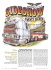fabricability and design considerations of heat resistant alloys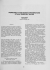Welcome to IF Metall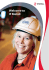Definition of Design Allowables for Aerospace Metallic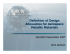Superplastic Behavior of an Extruded Mg-Zn-Y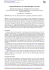PDF (Free)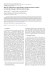Ambrotose® Products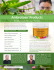processing and some applications of nickel, cobalt and titanium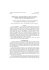Cenegenics Phoenix Micronutrient Testing Brochure